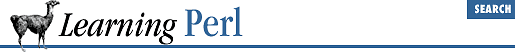home | O'Reilly's CD bookshelfs | FreeBSD | Linux | Cisco | Cisco Exam

### 5.4 Hash Functions

This section lists some functions for hashes.

### 5.4.1 The keys Function

The ``` keys(``` ``` %hashname ``` ``` )``` function yields a list of all the current keys in the hash ``` %hashname ``` . In other words, it's like the odd-numbered (first, third, fifth, and so on) elements of the list returned by unwinding ``` %hashname ``` in an array context, and in fact, returns them in that order. If there are no elements to the hash, then ``` keys``` returns an empty list.

For example, using the hash from the previous examples:

```\$fred{"aaa"} = "bbb";
\$fred{234.5} = 456.7;
@list = keys(%fred); # @list gets ("aaa",234.5) or
# (234.5,"aaa")```

As with all other built-in functions, the parentheses are optional: ``` keys ``` ``` %fred ``` is like ``` keys(``` ``` %fred ``` ``` )``` .

```foreach \$key (keys (%fred)) { # once for each key of %fred
print "at \$key we have \$fred{\$key}\n"; # show key and value
}```

This example also shows that individual hash elements can be interpolated into double-quoted strings. You cannot interpolate the entire hash, however.[ 3 ]

 Well, you can, using a slice, but we don't talk about slices here.

In a scalar context, the ``` keys``` function gives the number of elements (key-value pairs) in the hash. For example, you can find out whether a hash is empty:

```

if (keys(```

%somehash
```
)) { # if keys() not zero:
...; # array is non empty
}
# ... or ...

while (keys(```

%somehash
```
) < 10) {
...; # keep looping while we have fewer than 10 elements
} ```

In fact, merely using ``` %somehash ``` in a scalar context will reveal whether the hash is empty or not:

```if (```

%somehash
```
) { # if true, then something's in it
# do something with it
}```

### 5.4.2 The values Function

The ``` values(``` ``` %hashname ``` ``` )``` function returns a list of all the current values of the ``` %hashname ``` , in the same order as the keys returned by the ``` keys(``` ``` %hashname ``` ``` )``` function. As always, the parentheses are optional. For example:

```%lastname =

(); # force %lastname empty
\$lastname{"fred"} = "flintstone";
\$lastname{"barney"} = "rubble";
@lastnames = values(%lastname); # grab the values```

At this point ``` @lastnames``` contains either ``` ("flintstone"``` , ``` "rubble")``` or ``` ("rubble"``` , ``` "flintstone")``` .

### 5.4.3 The each Function

To iterate over (that is, examine every element of) an entire hash, use ``` keys``` , looking up each returned key to get the corresponding value. Although this method is frequently used, a more efficient way is to use ``` each(``` ``` %hashname ``` ``` )``` , which returns a key-value pair as a two-element list. On each evaluation of this function for the same hash, the next successive key-value pair is returned until all the elements have been accessed. When there are no more pairs, ``` each``` returns an empty list.

So, for example, to step through the ``` %lastname``` hash from the previous example, do something like this:

```

while ((\$first,\$last) = each(%lastname)) {
print "The last name of \$first is \$last\n";
}```

Assigning a new value to the entire hash resets the ``` each``` function to the beginning. Adding or deleting elements of the hash is quite likely to confuse ``` each``` (and possibly you as well).

### 5.4.4 The delete Function

So far, with what you know, you can add elements to a hash, but you cannot remove them (other than by assigning a new value to the entire hash). Perl provides the ``` delete``` function to remove hash elements. The operand of ``` delete``` is a hash reference, just as if you were merely looking at a particular value. Perl removes the key-value pair from the hash. For example:

```%fred = ("aaa","bbb",234.5,34.56); # give %fred two elements
delete \$fred{"aaa"};
# %fred is now just one key-value pair```5.3 Literal Representation of a Hash5.5 Hash Slices